### IMO Shortlist 1996 problem C5

Kvaliteta:
Avg: 0,0
Težina:
Avg: 8,0
Let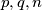$p,q,n$ be three positive integers with$p + q < n$. Let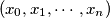$(x_{0},x_{1},\cdots ,x_{n})$ be an$(n + 1)$-tuple of integers satisfying the following conditions :

(a)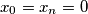$x_{0} = x_{n} = 0$, and

(b) For each$i$ with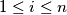$1\leq i\leq n$, either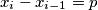$x_{i} - x_{i - 1} = p$ or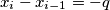$x_{i} - x_{i - 1} = - q$.

Show that there exist indices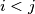$i < j$ with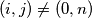$(i,j)\neq (0,n)$, such that$x_{i} = x_{j}$.
Izvor: Međunarodna matematička olimpijada, shortlist 1996